# Conservation Laws

## Concepts and Principles

### What is a Conservation Law?

In general, a conservation law is a statement that a certain quantity does not change over time. If you know how much of this quantity you have today, you can be assured that the exact same amount of the quantity will be available tomorrow. A famous (at least to physicists) explanation of the nature of a conservation law was given by Richard Feynman.

Imagine your child has a set of 20 wooden blocks. Every day before bedtime you gather up your child’s blocks to put them away. As you gather up the blocks, you keep count in your head. Once you reach 20, you know you have found all of the blocks and it is unnecessary for you to search any longer. This is because the number of blocks is conserved. It is the same today as it was yesterday.

If one day you only find 18 blocks, you know to keep looking until you find the missing 2 blocks. Also, with experience, you discover the typical hiding places for the blocks. You know to check under the sofa, or behind the curtains.

If your child is rambunctious, you may even have to look outside of the room. Perhaps he threw a block or two out of the window. Even though blocks can disappear from inside of the room, and appear out in the yard, if you search everywhere you will always find the 20 blocks.

Physicists have discovered a number of quantities that behave exactly like the number of wooden blocks. We will examine two of these quantities, energy and momentum, below.

### The Impulse-Momentum Relation

While Newton’s Second Law directly relates the total force that acts on an object at a specific time to the object’s acceleration at that exact same time, conservation laws relate the amount of a certain quantity present at one time to the amount present at a later time.

The first conserved quantity we will investigate is momentum. Of course, just because momentum is conserved doesn’t mean that the momentum of any particular object or system of objects is always constant. The momentum of a single object, like the number of blocks in the playroom, can change. Just as blocks can be thrown out of the window of the playroom, the momentum of a single object can be changed by applying impulse to it. The relationship between impulse and momentum is, conceptually,

initial momentum + impulse = final momentum

Pictorially, we can visualize this as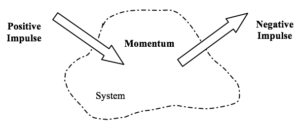In practice, we will identify an object or collection of objects (a system) and determine the amount of momentum the system contains at some initial time. This quantity cannot change unless impulse is done to the system. We call processes that bring momentum into the system as positive impulses, and processes that remove momentum from the system as negative impulses.

Mathematically this is written as

initial momentum + impulse = final momentum

Pi + Jif = Pf

where

• momentum (P) is the product of an object’s mass and velocity,
• impulse (J) is the product of a force external to the system and the time interval over which it acts,
• and S indicates that you must sum the momentum of all of the objects in the system and all of the impulses acting on the system.

In short, if no impulse is applied to a system, its momentum will remain constant. However, if an impulse is applied to the system, its momentum will change by an amount exactly equal to the impulse applied. This momentum does not appear or disappear without a trace. It is simply transferred to the object supplying the impulse. In this sense, impulse is the transfer of momentum into or out of a system, analogous to tossing blocks into or out of a playroom.

### The Work-Energy Relation

The second conserved quantity we will investigate is energy. Just like momentum, or wooden blocks, the conservation of energy doesn’t mean that the energy of any particular object is always constant. The energy of a single object or system of objects can be changed by doing work to it. The relationship between work and energy is, conceptually,

initial energy + work = final energy

Pictorially, we can visualize this as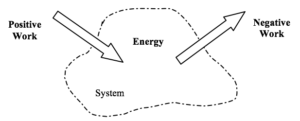The similarity between momentum and energy is not complete, however. While there is only one form of momentum (i.e., one hiding place for momentum “blocks”) there are several forms of energy. These different forms of energy will be introduced as you progress through more and more complicated models of the physical world. For now, the only “hiding place” I want to discuss is kinetic energy. In terms of kinetic energy, the above conceptual relationship between work and energy becomes, expressed mathematically,

initial energy + work = final energy

KEi + Wif = KEf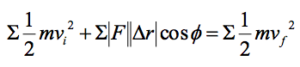where

• kinetic energy (KE) is the product of one-half an object’s mass and squared velocity,
• work (W) is the product of a force (even an internal force) and the displacement over which it acts (with more subtle details discussed below),
• S indicates that you must sum the kinetic energy of all of the objects in the system and all of the work done to the system,
• and we define a new unit, Joule (J), as J = kg (m/s)2 = N m.

Unlike anything we’ve studied up to this point, the work-energy relation is a scalar equation. This will become especially important when we study objects moving in more than one dimension. For now, all this means is that in the expression for work,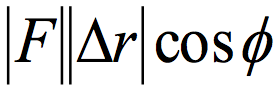, we should use the magnitude of the force and the magnitude of the change in position. This product is then multiplied by cos f , where f is defined to be the angle between the applied force and the displacement of the object. If the force and displacement are in the same direction f = 0°, and the work is positive (the object gains energy). If the force and displacement are in the opposite direction f = 180°, and the work is negative (the object loses energy). Note that the actual directions of the force and the displacement are unimportant, only their directions relative to each other affect the work.

In general, if no work is done to a system, its kinetic energy will remain constant. However, if work is done to system, its total energy will change by an amount exactly equal to the work done. Work is the transfer of energy from one system to another, again analogous to tossing blocks from the playroom into the yard.

## Analysis Tools

### Applying the Impulse-Momentum Relation to a Single Object

Let’s investigate the following scenario:

A 0.35 kg model rocket is fitted with an engine that produces a thrust of 11.8 N for 1.8 s. The rocket is launched vertically upward.

To apply the impulse-momentum relation, you must clearly specify the initial and final events at which you will tabulate the momentum. For example:

 Event 1: The instant the engine is ignited.   P1 = 0 Event 2: The instant the rocket reaches maximum height. P2 = (0.35) v2

J12 = +Fexhaust(1.8)-Fgravity(1.8)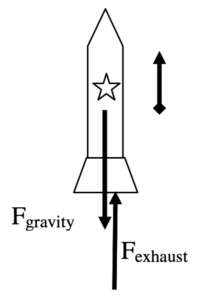Note that each external force acting on the rocket is multiplied by the time interval over which it acts. (Also note that the rocket’s engine does not produce a force on the rocket! The engine produces a downward force on the hot exhaust gases emitted from the engine and these hot gases exert an equal magnitude force back up on the rocket. That is why the force on the rocket is labeled as Fexhaust rather than Fengine.)

Applying impulse-momentum to the rocket during this time interval yields:

P1 + J12 = P2

0 + Fexhaust(1.8) – Fgravity(1.8) = 0.35v2

0 + (11.8)(1.8) – (0.35)(9.8)(1.8) = 0.35v2

v2 = 43.0 m/s

Thus, the rocket is traveling at 43.0 m/s at the instant the engine shuts off.
Of course, there is no reason why we had to analyze the rocket’s motion between the two instants of time we selected above. We could have selected the events:

 Event 1: The instant the engine is ignited.   P1 = Event 2: The instant the rocket reaches maximum height.   P2 = 0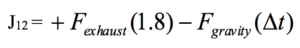During this time interval, the force of the exhaust gases only act on the rocket for a portion of the entire time interval. Noting that the rocket’s velocity when it reaches its maximum height is zero, impulse-momentum would look like this: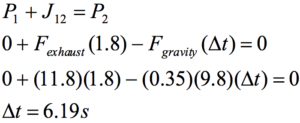Thus, the rocket is in the air for 6.19 s before reaching its maximum height.

### Applying the Work-Energy Relation to a Single Object

The work-energy relation also has many uses for investigating physical scenarios. For example, let’s look again at our model rocket:

A 0.35 kg model rocket is fitted with an engine that produces a thrust of 11.8 N for 1.8 s. The rocket is launched vertically upward.

Assuming we’ve already analyzed this scenario using impulse-momentum, what additional information can we extract using work-energy?

 Event 1: The instant the engine is ignited.   KE1 = 0 Event 2: The instant the engine shuts off. KE2 = ½ (0.35) (43)2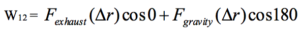Therefore,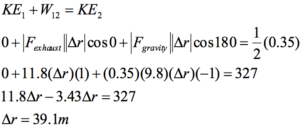What if we apply work-energy between the following two events:Thus, the rocket rises to a height of 39.1 m before the engines shuts off.

 Event 1: The instant the engine is ignited.   KE1 = 0 Event 2: The instant the rocket reaches its maximum height   KE2 = 0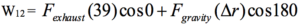During this time interval, the force of the exhaust gases only act on the rocket for a portion of the entire displacement, namely 39 m, while the force of gravity acts over the entire displacement.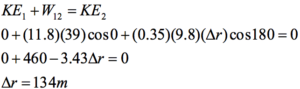Thus, the maximum height reached by the rocket is 134 m.

### Gravitational Potential Energy

In any situation in which an object changes its height above the surface of the earth, the force of gravity does work on the object. It is possible to calculate this work in general, and to rewrite the work-energy relation in such a way as to incorporate the effects of this work. This is referred to as constructing a potential energy function for the work done by gravity.

Let’s imagine an object of mass, m, located an initial height, hi, above the zero of a vertical coordinate system, with the upward direction designated positive. It moves to a final height of hf.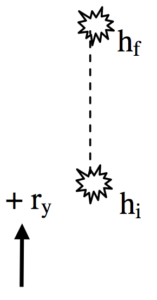To calculate the work done by gravity on this object: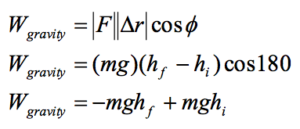The “mgh” terms are referred to as gravitational potential energy. Thus, the work done by gravity can be thought of as changing the gravitational potential energy of the object. Let’s plug the above result into the work-energy relation: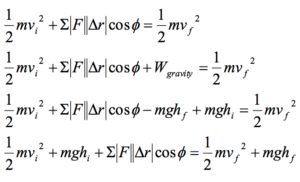Therefore, this final relation: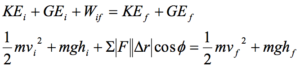can (and will) be used in place of the standard work-energy relation provided:

1. You do not include the force of gravity a second time by calculating the work done by gravity. Basically, in this relationship gravity is no longer thought of as a force that does work on objects but rather as a source of potential energy.
2. You calculate the initial and final heights, hi and hf, using a coordinate system in which the upward direction is positive.

### Applying Work-Energy with Gravitational Potential Energy

Let’s use the work-energy relation, with gravitational potential energy terms, to re-analyze the previous scenario:

A 0.35 kg model rocket is fitted with an engine that produces a thrust of 11.8 N for 1.8 s. The rocket is launched vertically upward.

Let’s apply work-energy between the following two events, setting the initial elevation of the rocket equal to zero:

 Event 1: The instant the engine is ignited.   KE1 = 0 GE1 = 0 Event 2: The instant the rocket reaches its maximum height   KE2 = 0 GE2 = (0.35)(9.8) h2

W12 = Fexhaust(39)cos0

During this time interval, the force of the exhaust gases only act on the rocket for a portion of the entire displacement, namely 39 m. Remember, the force of gravity does not do work in this way of modeling nature, rather the gravitational energy of the rocket changes as it changes its elevation.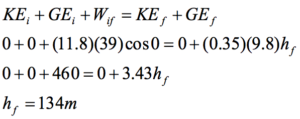results in, of course, the same maximum height reached by the rocket.

### Applying the Impulse-Momentum Relation to a Collision

Probably the most useful application of the impulse-momentum relation is in the study of collisions. For example:

Far from the earth, a 250 kg space probe, moving at 5 km/s, collides head-on with a 60 kg piece of space debris initially at rest. The debris becomes entangled in the probe’s solar collectors.

Let’s choose:

 Event 1: The instant before the collision.   Object: Space Probe   P1 = (250)(5000)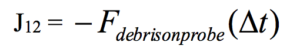Object: Debris   P1 = 0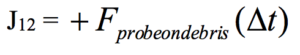Event 2: The instant the debris and probe reach a common velocity.       P2 = 250 v2         P2 = 60 v2

The free-body diagrams for the two objects during this time interval are shown below.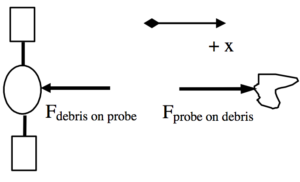Applying the impulse-momentum relation to each object separately yields: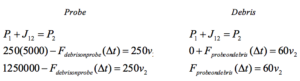Notice that the final velocities of the two objects are the same, because they remain joined together following the collision. Also, the Dt’s are the same because the time interval over which the force of the debris acts on the probe must be the same as the time interval over which the force of the probe acts on the debris. In fact, these two forces must be equal to each other in magnitude by Newton’s Third Law.

Thus, the impulses must cancel if the two equations are added together:The probe slows to a speed of 4032 m/s (and the debris changes direction and accelerates to a speed of 4032 m/s) via the collision. Thus, even though we do not know the magnitude of the force involved, or the duration of the collision, we can calculate the final velocities of the two objects colliding. This is because the forces involved comprise an interaction, and by Newton’s Third Law forces that comprise an interaction are always equal in magnitude and opposite in direction.

In fact, in problems involving collisions (or explosions, which to physicists are merely collisions played backward in time!), you should almost always apply the impulse-momentum relation to the interacting objects because the forces involved comprise an interaction. Thus, by adding your equations together, these terms will always add to zero. This will often allow you to determine the final velocities of the colliding objects.

In conclusion, I should point out that the probe loses momentum during the collision and that the debris gains the exact same amount of momentum. (Check the numbers to verify this statement.) The momentum is transferred from the probe to the debris through the action of the impulse the probe and debris exert on each other. The momentum transfer from the probe to the debris is analogous to throwing a wooden block from the playroom into the yard: The playroom now has one less block and the yard has one more!

### Applying the Work-Energy Relation to the Same Collision

Let’s return to the collision scenario discussed above and attempt to investigate it using work-energy.

Far from the earth, a 250 kg space probe, moving at 5 km/s, collides head-on with a 60 kg piece of space debris initially at rest. The debris becomes entangled in the probe’s solar collectors.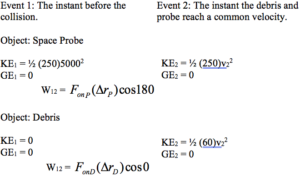Applying the work-energy relation to each object separately yields: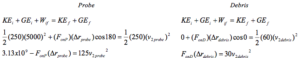The final velocities of the two objects are the same, because they remain joined together following the collision, and the two forces are the same by Newton’s Third Law. However, these two equations cannot be added together and solved because the two distances over which the forces act, Drprobe and Drdebris, are not necessarily equal. During the collision, the center of the probe will move a different distance that the center of the debris. Since these two distances are different, the works will not cancel as the impulses did, and the equations are not solvable!

In fact, since we know v2 = 4032 m/s from our momentum analysis,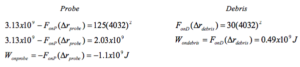Obviously, the two works do not cancel. In fact, the internal work, or work done by the objects on each other, totals -0.61 x 109 J. This means that there is 0.61 x 109 J less kinetic energy in the system of the probe and the debris after the collision than before the collision. This is sometimes referred to as the energy lost in the collision, although the energy is not lost but rather converted into other forms of energy (i.e., other hiding places for the wooden blocks that have yet to be discussed), such as thermal energy.

In short, the work-energy relation (as it now stands) cannot be used to effectively analyze collisions unless additional information regarding the internal energy is available. Occasionally, an approximation is made in which the total internal work is zero. When this approximation is made, the collision is referred to as an elastic collision. Realistic collisions, in which the total internal energy is not zero and kinetic energy is “lost”, are referred to as inelastic collisions.

## Activities

Below are momentum vs. time graphs for six different objects.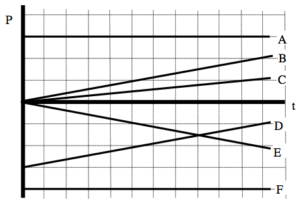a. Rank these graphs on the basis of the change in momentum of the object over the time interval indicated.

Largest Positive  1. _____ 2. _____ 3. _____ 4. _____ 5. _____ 6. _____ Largest Negative

_____ The ranking cannot be determined based on the information provided.

Explain the reason for your ranking:

b. Rank these graphs on the basis of the total impulse on the object over the time interval indicated.

Largest Positive  1. _____ 2. _____ 3. _____ 4. _____ 5. _____ 6. _____ Largest Negative

_____ The ranking cannot be determined based on the information provided.

Explain the reason for your ranking:

Below are kinetic energy vs. time graphs for six different objects. All of the objects move horizontally.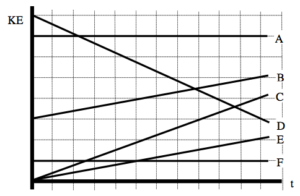Rank these graphs on the basis of the change in kinetic energy of the object over the time interval indicated.

Largest Positive  1. _____ 2. _____ 3. _____ 4. _____ 5. _____ 6. _____ Largest Negative

_____ The ranking cannot be determined based on the information provided.

Explain the reason for your ranking:

Rank these graphs on the basis of the total work on the object over the time interval indicated.

Largest Positive  1. _____ 2. _____ 3. _____ 4. _____ 5. _____ 6. _____ Largest Negative

_____ The ranking cannot be determined based on the information provided.

Explain the reason for your ranking:

Below are velocity vs. time graphs for six equal-mass objects.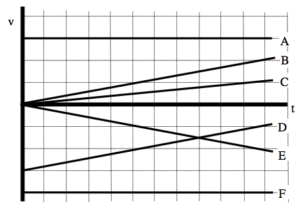Rank these graphs on the basis of the change in momentum of the object over the time interval indicated.

Largest Positive  1. _____ 2. _____ 3. _____ 4. _____ 5. _____ 6. _____ Largest Negative

_____ The ranking cannot be determined based on the information provided.

Explain the reason for your ranking:

Rank these graphs on the basis of the change in kinetic energy of the object over the time interval indicated.

Largest Positive  1. _____ 2. _____ 3. _____ 4. _____ 5. _____ 6. _____ Largest Negative

_____ The ranking cannot be determined based on the information provided.

Explain the reason for your ranking:

Below are velocity vs. time graphs for six equal-mass objects. All of the objects move horizontally.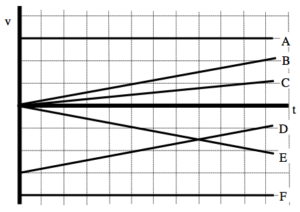Rank these graphs on the basis of the total impulse on the object over the time interval indicated.

Largest Positive  1. _____ 2. _____ 3. _____ 4. _____ 5. _____ 6. _____ Largest Negative

_____ The ranking cannot be determined based on the information provided.

Explain the reason for your ranking:

Rank these graphs on the basis of the total work on the object over the time interval indicated.

Largest Positive  1. _____ 2. _____ 3. _____ 4. _____ 5. _____ 6. _____ Largest Negative

_____ The ranking cannot be determined based on the information provided.

Explain the reason for your ranking:

Below are six automobiles initially traveling at the indicated velocity. The automobiles have different masses and velocities.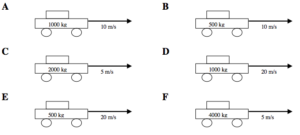All automobiles will be stopped in the same amount of time. Rank these automobiles on the basis of the magnitude of the force needed to stop them.

Largest  1. _____ 2. _____ 3. _____ 4. _____ 5. _____ 6. _____ Smallest

_____ The ranking cannot be determined based on the information provided.

Explain the reason for your ranking:

All automobiles will be stopped in the same amount of distance. Rank these automobiles on the basis of the magnitude of the force needed to stop them.

Largest  1. _____ 2. _____ 3. _____ 4. _____ 5. _____ 6. _____ Smallest

_____ The ranking cannot be determined based on the information provided.

Explain the reason for your ranking:

Below are six automobiles initially traveling at the indicated velocity. The automobiles have different masses and velocities.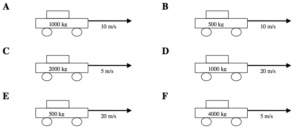Rank these automobiles on the basis of the magnitude of the force needed to stop them.

Largest  1. _____ 2. _____ 3. _____ 4. _____ 5. _____ 6. _____ Smallest

_____ The ranking cannot be determined based on the information provided.

Explain the reason for your ranking:

Rank these automobiles on the basis of the magnitude of the work needed to stop them.

Largest  1. _____ 2. _____ 3. _____ 4. _____ 5. _____ 6. _____ Smallest

_____ The ranking cannot be determined based on the information provided.

Explain the reason for your ranking:

Rank these automobiles on the basis of the magnitude of the impulse needed to stop them.

Largest  1. _____ 2. _____ 3. _____ 4. _____ 5. _____ 6. _____ Smallest

_____ The ranking cannot be determined based on the information provided.

Explain the reason for your ranking:

For each of the collisions illustrated below, sketch a graph of the momentum and velocity of asteroid A, the momentum and velocity of asteroid B, and the total momentum in the system of the two asteroids. Begin your graph before the collision takes place and continue it after the collision is over. Use a consistent scale on all graphs.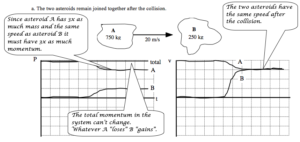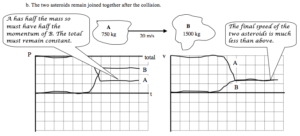For each of the collisions illustrated below, sketch a graph of the momentum and velocity of asteroid A, the momentum and velocity of asteroid B, and the total momentum in the system of the two asteroids. Begin your graph before the collision takes place and continue it after the collision is over. Use a consistent scale on all graphs.

a. The two asteroids remain joined together after the collision.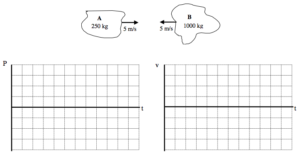b. The two asteroids remain joined together after the collision.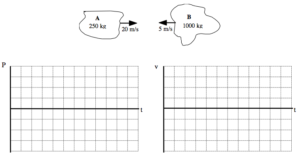For each of the collisions illustrated below, sketch a graph of the momentum and velocity of asteroid A, the momentum and velocity of asteroid B, and the total momentum in the system of the two asteroids. Begin your graph before the collision takes place and continue it after the collision is over. Use a consistent scale on all graphs.

a. The two asteroids remain joined together after the collision.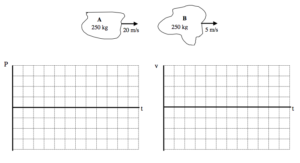b. The two asteroids remain joined together after the collision.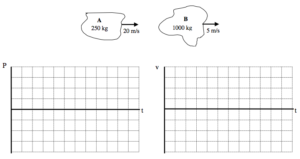For each of the collisions illustrated below, sketch a graph of the momentum and velocity of asteroid A, the momentum and velocity of asteroid B, and the total momentum in the system of the two asteroids. Begin your graph before the collision takes place and continue it after the collision is over. Use a consistent scale on all graphs.

a. Asteroid A rebounds at 5 m/s after the collision.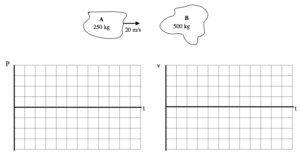b. Asteroid B moves at 20 m/s after the collision.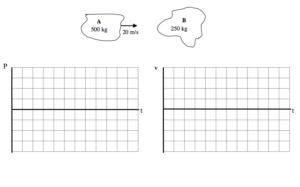For each of the explosions illustrated below, sketch a graph of the momentum and velocity of fragment A, the momentum and velocity of fragment B, and the total momentum in the system of the two fragments. Begin your graph before the explosion takes place and continue it while the fragments travel away from the sight of the explosion. Use a consistent coordinate system and scale on all graphs.

a. The exploding egg is initially at rest.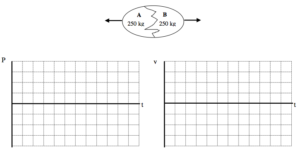b. The exploding egg is initially at rest.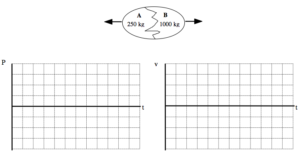A 200 kg astronaut is initially at rest on the extreme edge of a 1000 kg space platform. She wears special magnetic shoes that allow her to walk along the metal platform. For each of the situations illustrated below, sketch a graph of the momentum and velocity of the astronaut, the momentum and velocity of the platform, and the total momentum in the system of the two objects. Begin your graph before the astronaut begins to walk and continue it while she walks along the platform. Use a consistent coordinate system and scale on all graphs.

a. The astronaut and platform are initially at rest.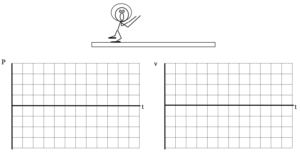b. The astronaut and platform are initially drifting to the right.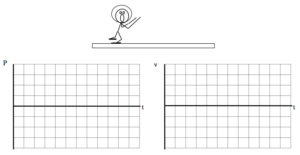For each of the scenarios described below, indicate the amount of kinetic energy and gravitational potential energy of the object at each of the events listed. Use a consistent scale throughout each motion. Set the lowest point of the motion as the zero-point of gravitational potential energy

a. A 4000 kg rocket’s engine produces a thrust of 70,000 N for 15 s. The rocket is fired vertically upward.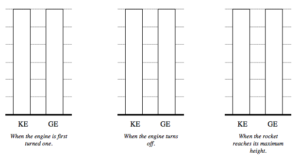b. To practice falling, a 55 kg pole-vaulter falls off of a wall 6.0 m above a 2.0 m thick foam cushion resting on the ground. However, he misses the cushion. The pole-vaulter sinks about 0.10 m into the ground before stopping.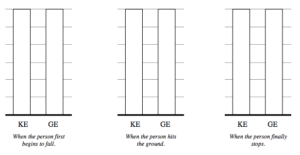For each of the scenarios described below, indicate the amount of kinetic energy and gravitational potential energy of each object at each of the events listed. Use a consistent scale throughout each motion. Set the lowest point of the motion as the zero-point of gravitational potential energy

a. Tired of walking up the stairs, an 80 kg engineering student designs an ingenious device for reaching his third floor dorm room. An 84 kg block is attached to a rope that passes over a pulley. The student holds the other end of the rope. When the 84 kg block is released, the student is pulled up to his dorm room, 8.0 m off the ground.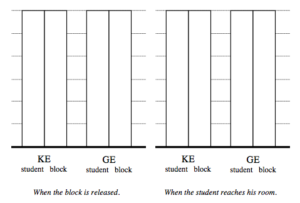b. Tired of walking down the stairs, a 75 kg engineering student designs an ingenious device for reaching the ground from his third floor dorm room. A 60 kg block at rest on the ground is attached to a rope that passes over a pulley. The student grabs the other end of the rope and steps out of his window.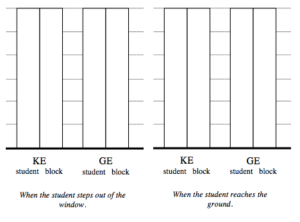For the following problems,

A 100 kg man concerned about his weight decides to weigh himself in an elevator. He stands on a bathroom scale in an elevator that is moving upward at 3.0 m/s. As the elevator reaches his floor, it slows to a stop.

a. If the elevator slows to a stop over a distance of 2.0 m, what is the reading on the bathroom scale?

b. If the elevator slows to a stop in 1.5 s, what is the reading on the bathroom scale?

 Motion Diagram a. Motion Information Event 1:   KE1 = GE1 = W12 = Event 2:   KE2 = GE2 = Mathematical Analysis[i]

 Free-Body Diagram b. Motion Information Event 1:   P1 = J12 = Event 2:   P2 = Mathematical Analysis

A 70 kg student is 120 m above the ground, moving upward at 3.5 m/s, while hanging from a rope hanging from a 280 kg helium balloon. The lift on the balloon due to the buoyant force is 3000 N.

a. With what speed does the student hit the ground?

b. How long does it take the student to reach the ground?

 Motion Diagram a. Motion Information Event 1:   KE1 = GE1 = W12 = Event 2:   KE2 = GE2 = Mathematical Analysis[ii]

 Free-Body Diagram   student & balloon b. Motion Information Event 1:   P1 = J12 = Event 2:   P2 = Mathematical Analysis

A 4000 kg rocket’s engine produces a thrust of 70,000 N for 15 s. The rocket is fired vertically upward.

a. What is the speed of the rocket when its engine turns off?

b. How long does it take the rocket to reach its maximum height?

 Motion Diagram a. Motion Information Event 1:   P1 = J12 = Event 2:   P2 = Mathematical Analysis[iii]

 Free-Body Diagrams   while engine fires       after engine turns off b. Motion Information Event 1:   P1 = J12 = Event 2:   P2 = Mathematical Analysis

To practice falling, a 55 kg pole-vaulter falls off of a wall 6.0 m above a 2.0 m thick foam cushion resting on the ground. However, he misses the cushion. The pole-vaulter sinks about 0.10 m into the ground before stopping.

a. What is the speed of the pole-vaulter when he hits the ground?

b. What is the force exerted on the pole-vaulter by the ground as he comes to rest?

 Motion Diagram a. Motion Information Event 1:   KE1 = GE1 = W12 = Event 2:   KE2 = GE2 = Mathematical Analysis[iv]

 Free-Body Diagrams   while falling       while dying b. Motion Information Event 1:   KE1 = GE1 = W12 = Event 2:   KE2 = GE2 = Mathematical Analysis

A decorative light fixture in an elevator consists of a 2.0 kg light suspended by a cable from the ceiling of the elevator. From this light, a separate cable suspends a second 0.80 kg light. The elevator is moving downward at 4.0 m/s when someone presses the emergency stop button. During the stop, the upper cable snaps. The elevator engineer says that the cable could withstand a force of 40 N without breaking. Find the maximum time and distance over which the elevator stopped.

 Motion Diagram Motion Information Event 1:   P1 = J12 = KE1 = GE1 = W12 = Event 2:   P2 =   KE2 = GE2 = Mathematical Analysis[v]

 Free-Body Diagrams   the two lights

Tired of walking up the stairs, an 80 kg engineering student designs an ingenious device for reaching his third floor dorm room. An 84 kg block is attached to a rope that passes over a pulley. The student holds the other end of the rope. When the 84 kg block is released, the student is pulled up to his dorm room, 8.0 m off the ground.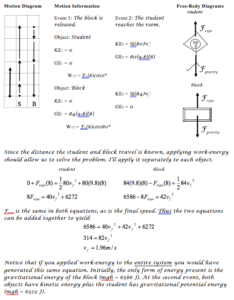Tired of walking up the stairs, an engineering student designs an ingenious device for reaching his third floor dorm room. A 100 kg block is attached to a rope that passes over a pulley. The student holds the other end of the rope. When the block is released, the student is pulled up to his dorm room, 8.0 m off the ground. He is traveling at 2.2 m/s when he reaches his room.

 Motion Diagram Motion Information Event 1:   Object: KE1 = GE1 = W12 = Object: KE1 = GE1 = W12 = Event 2:     KE2 = GE2 =     KE2 = GE2 = Free-Body Diagrams              student                 block

 Mathematical Analysis[vi]

Tired of walking down the stairs, a 75 kg engineering student designs an ingenious device for reaching the ground from his third floor dorm room. A 60 kg block at rest on the ground is attached to a rope that passes over a pulley. The student grabs the other end of the rope and steps out of his window. He falls for 5.5 s before reaching the ground.

 Motion Diagram Motion Information Event 1:   Object: P1 = J12 = Object: P1 = J12 Event 2:     P2 =     P2 = Free-Body Diagrams              student                 block

 Mathematical Analysis[vii]

Tired of walking down the stairs, a 75 kg engineering student designs an ingenious device for reaching the ground from his dorm room. A 60 kg block at rest on the ground is attached to a rope that passes over a pulley. The student grabs the other end of the rope and steps out of his window. He hits the ground at 3.3 m/s.

 Motion Diagram Motion Information Event 1: Object: P1 = J12 = KE1 = GE1 = W12 = Event 2:   P2 =   KE2 = GE2 = Object: P1 = J12 = KE1 = GE1 = W12 = P2 =   KE2 = GE2 =

 Free-Body Diagrams           student                block Mathematical Analysis[viii]

Far from any other masses, a 2000 kg asteroid traveling at 12 m/s collides with a 1200 kg asteroid traveling in the other direction at 16 m/s. After the collision they remain joined together and move with a common velocity.

 Motion Diagram Free-Body Diagrams   2000 kg asteroid 1200 kg asteroid Motion Information Event 1:   Object: P1 = J12 = Object: P1 = J12 = Event 2:     P2 =     P2 = Mathematical Analysis[ix]

On a remote stretch of intergalactic highway, a 7.5 x 106 kg spaceship traveling at 10 percent the speed of light (0.10c = 3.0 x 107 m/s) doesn’t notice the slower spaceship ahead clogging the lane. The fast-moving ship rear-ends the slower ship, an older 5.5 x 106 kg model, and the two ships get entangled and drift forward at 0.07c.

 Motion Diagram Free-Body Diagrams   fast ship slow ship Motion Information Event 1:   Object: P1 = J12 = Object: P1 = J12 = Event 2:     P2 =     P2 = Mathematical Analysis[x]

On a remote stretch of intergalactic highway, a 7.5 x 106 kg spaceship traveling at 10 percent the speed of light (0.10c = 3.0 x 107 m/s) doesn’t notice the slower spaceship ahead, moving at 0.05c, clogging the lane. The fast-moving ship rear-ends the slower ship, an older 4.5 x 106 kg model, and the slower ship gets propelled forward at 0.13c.

 Motion Diagram Free-Body Diagrams   fast ship slow ship Motion Information Event 1:   Object: P1 = J12 = Object: P1 = J12 = Event 2:     P2 =     P2 = Mathematical Analysis[xi]

In the farthest reaches of deep space, an 8000 kg spaceship, including contents, is at rest relative to a space station. The spaceship recoils after it launches a 600 kg scientific probe with a speed of 300 m/s relative to the space station.

 Motion Diagram Free-Body Diagrams   spaceship probe Motion Information Event 1:   Object: P1 = J12 = Object: P1 = J12 = Event 2:     P2 =     P2 = Mathematical Analysis[xii]

In the farthest reaches of deep space, an 8000 kg spaceship, including contents, is drifting at 50 m/s relative to a space station. The spaceship is brought to rest, relative to the space station, by the recoil from launching a 600 kg scientific probe.

 Motion Diagram Free-Body Diagrams   spaceship probe Motion Information Event 1:   Object: P1 = J12 = Object: P1 = J12 = Event 2:     P2 =     P2 = Mathematical Analysis[xiii]

A 140 kg astronaut is standing on the extreme edge of a 1000 kg space platform, at rest relative to the mother ship. She begins to walk toward the other edge of the platform, reaching a speed of 2.0 m/s relative to the mother ship. (She wears special magnetic shoes that allow her to walk along the metal platform.)

 Motion Diagram Free-Body Diagrams   astronaut platform Motion Information Event 1:   Object: P1 = J12 = Object: P1 = J12 = Event 2:     P2 =     P2 = Mathematical Analysis[xiv]

Two astronauts, 140 kg Andy and 170 kg Bob, are standing on opposite edges of a 1000 kg space platform, at rest relative to the mother ship. They each begin to walk toward the opposite ends of the platform, Andy reaching a speed of 2.0 m/s and Bob 1.5 m/s, both relative to the mother ship. (They wear special magnetic shoes that allow them to walk along the metal platform.)

Motion Diagram

Free-Body Diagrams

 Andy platform Bob Motion Information Event 1:   Object: P1 = J12 = Object: P1 = J12 = Object: P1 = J12 = Event 2:     P2 =     P2 =     P2 = Mathematical Analysis[xv]

A 70 kg student is hanging from a 280 kg helium balloon. The balloon is rising at a constant speed of 8.0 m/s relative to the ground. The lift on the balloon due to the buoyant force is constant. The student begins to climb up the rope at a speed of 15 m/s relative to the ground. The balloon’s upward speed is decreased as the student climbs.

 Motion Diagram Motion Information Event 1:   Object: P1 = J12 = Object: P1 = J12 = Event 2:     P2 =     P2 = Free-Body Diagrams   student   balloon   Mathematical Analysis

A man of mass m, concerned about his weight, decides to weigh himself in an elevator. He stands on a bathroom scale in an elevator which is moving upward at v. As the elevator reaches his floor, it slows to a stop over a time interval, T. Determine the reading on the bathroom scale (Fscale) as a function of m, v, T, and g.

 Motion Diagram Motion Information Event 1:   P1 = J12 = Event 2:   P2 = Free-Body Diagram

Questions

If T = ∞, what should Fscale equal? Does your function agree with this observation?

For what combination of v and T would the bathroom scale read 0 N?

If the elevator were initially going down, would the above combination of v and T also lead to a scale reading of 0 N?

A rocket of mass m is fired vertically upward from rest. The rocket’s engine produces a thrust of constant magnitude F for tthrust seconds. Determine the time it takes the rocket to reach its apex (tapex) as a function of F, tthrust, m, and g.

 Motion Diagram Motion Information Event 1:   P1 = J12 = Event 2:   P2 = Free-Body Diagram

Questions

If g = 0 m/s2, what should tapex equal? Does your function agree with this observation?

If F = mg, what should tapex equal? Does your function agree with this observation?

For what value of F would tapex = 2tthrust?

To practice falling, a pole-vaulter of mass m falls off of a wall a distance D above a thick foam cushion. The pole-vaulter sinks a distance d into the cushion before stopping. Determine the force exerted on the pole-vaulter due to the cushion (Fcushion) as a function of m, D, d, and g.

 Motion Diagram Motion Information Event 1:   KE1 = GE1 = W12 = Event 2:   KE2 = GE2 = Free-Body Diagrams   while falling         while dying

Questions

If D = ∞, what should Fcushion equal? Does your function agree with this observation?

If d = 0 m, what should Fcushion equal? Does your function agree with this observation?

What would be worse for the pole-vaulter, starting at twice the initial distance above the cushion or sinking half of the original distance into the cushion?

Tired of walking up the stairs, an engineering student of mass m designs an ingenious device for reaching his third floor dorm room. A block of mass M is attached to a rope that passes over a pulley. The student holds the other end of the rope. When the block is released, the student is pulled up to his dorm room in a time T. Determine the velocity of the student (v) when he reaches his room as a function of m, M, T and g.

Motion Diagram    Motion Information                                                                                          Free-Body Diagrams

 Event 1:   Object: P1 = J12 = Object: P1 = J12 = Event 2:     P2 =     P2 = student   block

Questions

If g = 0 m/s2, what should v equal? Does your function agree with this observation?

If m = M, what should v equal? Does your function agree with this observation?

If M = ∞, what should v equal? Does your function agree with this observation?

Tired of walking down the stairs, an engineering student of mass m designs an ingenious device for reaching the ground from her dorm room. A block of mass M at rest on the ground is attached to a rope that passes over a pulley. The student grabs the other end of the rope and steps out of her window a distance D above the ground. Determine the velocity of the student (v) when she reaches the ground as a function of m, M, D and g.

Motion Diagram    Motion Information                                                                                                    Free-Body Diagrams

 Event 1: Object: KE1 = GE1 = W12 = Object: KE1 = GE1 = W12 = Event 2:   KE2 = GE2 =     KE2 = GE2 = student   Block

Questions

If g = 0 m/s2, what should v equal? Does your function agree with this observation?

If m = M, what should v equal? Does your function agree with this observation?

If M = ∞, what should v equal? Does your function agree with this observation?

In the farthest reaches of deep space, a spaceship of mass M, including contents, is at rest relative to a space station. The spaceship recoils after it launches a scientific probe of mass m at a speed v relative to the space station. Determine the recoil speed of the spaceship (V) as a function of M, m, and v.

 Motion Diagram Free-Body Diagrams   spaceship probe Motion Information Event 1:   Object: P1 = J12 = Object: P1 = J12 = Event 2:     P2 =     P2 =

Questions

If M = 2m, what should V equal? Does your function agree with this observation?

If M = ∞, what should V equal? Does your function agree with this observation?

 If the two objects were actually particles, rather than being approximated as particles, then the two distances would have to be the same and the two works would cancel when the equation were added together.

[i] a. F scale = 755 N               b. F scale = 780 N

[ii] a. v = 17.5 m/s                               b. t = 17.1 s

[iii] a. v = 116 m/s                               b. t = 26.8 s

[iv] a. v = 12.5 m/s                              b. F ground = 43700 N

[v] t2 = 0.89 s                                     r2 = 1.78 m

[vi] m student = 94 kg

[vii] v2 = 6.0 m/s

[viii] Student falls 5.0 m in 3.03 s

[ix] v2 = 1.5 m/s

[x] v1 slowship = 0.029c = 8.73 x 106 m/s

[xi] v2 fastship = 0.052c = 1.56 x 107 m/s

[xii] v2 ship = 24.3 m/s

[xiii] v probe = 667 m/s

[xiv] v2 platform = 0.28 m/s

[xv] v2 platform = 0.025 m/s

[xvi] v2 balloon = 6.3 m/s

Homework 3 – Model 1: 117, 118, 120, 121, 127, 131, 134, 138, 144, and 147.

1. If the two objects were actually particles, rather than being approximated as particles, then the two distances would have to be the same and the two works would cancel when the equation were added together.